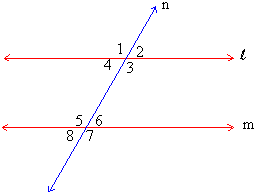Home MonkeyNotes Printable Notes Digital Library Study Guides Message Boards Study Smart Parents Tips College Planning Test Prep Fun Zone Help / FAQ How to Cite New Title Request

 1.6 Transversal across two parallel lines Corresponding Angles : If a transversal cuts two parallel lines the corresponding angles are equal ( figure 1.26 )Figure 1.26 l & m are two parallel lines cut by a transversal n to form angles 1 to 8. Axiom : Corresponding angles are equal in measure if a transversal cuts parallel lines. m Ð 1 = m Ð 5 m Ð 2 = m Ð 6 m Ð 3 = m Ð 7 m Ð 4 = m Ð 8 . Alternate interior angles : If a transversal cuts two parallel lines the alternate interior angles are equal in measure. In figure 1.26 m Ð 4 = m Ð 6 and m Ð 3 = m Ð 5. This can be proved as follows : m Ð 3 = m Ð 4 are supplementary and so also m Ð 6 = m Ð 7 are supplementary. Since the sums of their measures are 1800 in both cases. m Ð 3 + m Ð 4 = m Ð 6 + m Ð 7. However m Ð 3 = m Ð 7 as they are corresponding angles formed by a transversal across parallel lines. Therefore, m Ð 3 + m Ð 4 = m Ð 6 + m Ð 3 i.e. m Ð 4 = m Ð 6. Similarly it can be shown that m Ð 3 = m Ð 5 . Alternate exterior angles : If a transversal cuts two parallel lines the alternate exterior angles are equal in measure. In figure 1.26 m Ð 1= m Ð 7 and m Ð 2 = m Ð 8. This can be easily shown as follows : m Ð 1 = m Ð 3 (vertical angles) m Ð 3 = m Ð 7 (corresponding angles on parallel lines) \ mÐ 1 = m Ð 7 Consecutive Interior and Consecutive Exterior Angles : If a transversal cuts two parallel lines the consecutive interior angles and consecutive exterior angles are supplementary. In figure 1.26 m Ð 4 and m Ð 5 are supplementary and also m Ð 3 and m Ð 6 are supplementary. This is proven as : m Ð 1 and m Ð 4 are supplementary but m Ð 1 = m Ð 5 (corresponding angles on parallel lines ) \ m Ð 4 and m Ð 5 are supplementary. Since consecutive exterior angles are supplementary. m Ð 1 and m Ð 8 so also m Ð 2 and m Ð 7 should be supplementary. Proof : m Ð 1 and m Ð 4 are supplementary but m Ð 4 = m Ð 8 (corresponding angles on parallel lines ) \ m Ð 1 and m Ð 8 are supplementary. Index Introduction 1.1 Points, Lines and Planes 1.2 Line Segment 1.3 Rays and Angles 1.4 Some Special Angles 1.5 Angles made by a Transversal 1.6 Transversal Across Two Parallel Lines 1.7 Conditions for Parallelism Chapter 2
 Search: All Products Books Popular Music Classical Music Video DVD Toys & Games Electronics Software Tools & Hardware Outdoor Living Kitchen & Housewares Camera & Photo Cell Phones Keywords: